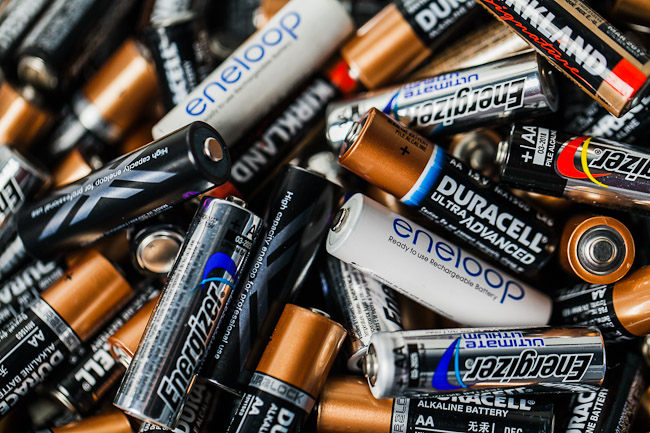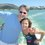# Battery OptimizationHi all,

Suppose we have $n$ batteries, $g$ good ones, and a flashlight that takes $c$ batteries. And you don't know which are the bad ones.

And, let $f(n,g,c) =$ The minimum number of attempts (insertions of $c$ batteries into the flashlight) you need in order to get your flashlight working.

And assume that the only way the flashlight will work is if all $c$ batteries you put in the flashlight are good. Otherwise, it won't work, and there is no way to distinguish between any combination that contains one or more bad batteries.

What I am wondering is how to show/prove the general solution for $f(n,g,c)$.

We have a few trivial cases:

• $f(n,g,1) = n - g +1$
• $f(n,g,g) = \binom{n}{g}$
• $f(n,n,c) = 1$
• $f(4,3,2) = 2$

So, I think the first non-trivial case becomes:

• $f(5,3,2)$

This one can be done in four moves, (1 vs 2, 3 vs 4, 4 vs 5, 3 vs 5) but can we prove that it can't be done in fewer?

And, does anyone have any idea about how we can solve this problem for the general case?

Thanks!

GeoffNote by Geoff Pilling
4 years, 3 months ago

This discussion board is a place to discuss our Daily Challenges and the math and science related to those challenges. Explanations are more than just a solution — they should explain the steps and thinking strategies that you used to obtain the solution. Comments should further the discussion of math and science.

When posting on Brilliant:

• Use the emojis to react to an explanation, whether you're congratulating a job well done , or just really confused .
• Ask specific questions about the challenge or the steps in somebody's explanation. Well-posed questions can add a lot to the discussion, but posting "I don't understand!" doesn't help anyone.
• Try to contribute something new to the discussion, whether it is an extension, generalization or other idea related to the challenge.
• Stay on topic — we're all here to learn more about math and science, not to hear about your favorite get-rich-quick scheme or current world events.

MarkdownAppears as
*italics* or _italics_ italics
**bold** or __bold__ bold
- bulleted- list
• bulleted
• list
1. numbered2. list
1. numbered
2. list
Note: you must add a full line of space before and after lists for them to show up correctly
paragraph 1paragraph 2

paragraph 1

paragraph 2

[example link](https://brilliant.org)example link
> This is a quote
This is a quote
    # I indented these lines
# 4 spaces, and now they show
# up as a code block.

print "hello world"
# I indented these lines
# 4 spaces, and now they show
# up as a code block.

print "hello world"
MathAppears as
Remember to wrap math in $$ ... $$ or $ ... $ to ensure proper formatting.
2 \times 3 $2 \times 3$
2^{34} $2^{34}$
a_{i-1} $a_{i-1}$
\frac{2}{3} $\frac{2}{3}$
\sqrt{2} $\sqrt{2}$
\sum_{i=1}^3 $\sum_{i=1}^3$
\sin \theta $\sin \theta$
\boxed{123} $\boxed{123}$

## Comments

Sort by:

Top Newest

@Brian Charlesworth In a previous thread you had suggested that for $f(n,3,2)$ you might want to split the group into two, one of which is guaranteed to have two good batteries, and run through each of the combinations in each group, giving:

$f(2n,3,2) = 2\dbinom{n}{2}$ and $f(2n + 1,3,2) = \dbinom{n}{2} + \dbinom{n + 1}{2}$

One generalization of that for $g>3$ might be to split up the batteries into the greatest number of groups ($g-1$?) such that at least one is guaranteed to have at least two good batteries and then go through all the combinations in each group. Without showing that this is the best approach, it certainly seems like a promising one.

So, for example if we take $g=4$, then we would split into 3 groups, yielding:

$f(8,4,2) = \binom{2}{2} + \binom{3}{2} + \binom{3}{2} = 1 + 3 + 3 = 7$

So for $f(100,50,2)$ we would have:

$f(100,50,2) = 2\binom{3}{2} + 47\binom{2}{2} = 53$

Recognize that answer? :0)

A couple more examples using the above algorithm gives:

• $f(100,10,2) = 8\binom{11}{2} + \binom{12}{2} = 506$
• $f(100,25,2) = 4\binom{5}{2} + 20\binom{4}{2} = 160$

@Calvin Lin Thoughts on how one might go about proving that these could be optimal approaches (outside of using a computer)?

- 4 years, 3 months ago

Log in to reply

Once you summarize the approach it does look quite promising. :)

As far as I can tell, with $f(n,g,2)$ your goal is to form the sum

$a\dbinom{\lfloor\frac{n}{g - 1}\rfloor}{2} + b\dbinom{\lfloor\frac{n}{g - 1}\rfloor + 1}{2}$

so as to maximize $a$ given $a + b = g - 1$.

I can see one other approach to get $f(100,10,2) = 506$, where we have $9$ groupings of $11$ and one singleton left over. Then if after $9\binom{11}{2} = 495$ tests with no success we can conclude that the singleton is good and that each of the $9$ groups has one good battery as well. So we then choose one of these groups and pair all $11$ in the group with the good singleton to finally get a working pair after a grand total of $495 + 11 = 506$ trials. This isn't an improvement but it does suggest that the $g - 1$ paradigm may not be exclusively optimal.

- 4 years, 3 months ago

Log in to reply

Without a proof, I suspect that the general solution for $f(n,g,c)$ might be given by this:

1) Divide the $n$ batteries up into the maximum number of groups you can (minimizing the maximum number for any group) so that at least one group contains at least $c$ batteries. I think this number is given by:

• Number of groups $= \lfloor {\frac{g-1}{c-1}} \rfloor$

2) Perform all possible iterations within each group.

So, for example, for $f(100,50,3)$ you would split it up into $20$ groups of $4$ and $4$ groups of $5$, guaranteeing that at least one group has $3$.

$\implies f(100,50,3) = 20\binom{4}{3} + 4\binom{5}{3}$

- 4 years, 3 months ago

Log in to reply

That looks good. The values ramp up quite quickly with $c$, with

$f(100,50,4) = 12\binom{6}{4} + 4\binom{7}{4} = 320, f(100,50,5) = 8\binom{8}{5} + 4\binom{9}{5} = 952$

and $f(100,50,6) = 8\binom{11}{6} + \binom{12}{6} = 4620$.

We could probably write out a messy general formula now but I think the description of the process suffices. The optimization proof remains elusive, however ...

- 4 years, 3 months ago

Log in to reply

Yes. Looks like a good generalization/summary for $c=2$... Now we just gotta figure out a proof and/or generalize it for $c>2$... Hmmmm....

- 4 years, 3 months ago

Log in to reply

×

Problem Loading...

Note Loading...

Set Loading...